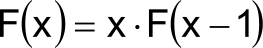Abstract

In the article will be shown that factorial can be computed by the following formula:

(30)Gamma constant can be defined by the following formula:

(27)Gamma constant has following numerical value γ = 0.57721566490153286060651209008.

Press the following button to download the article in the PDF format:

A REPRESENTATION OF FACTORIAL FUNCTION, THE NATURE OF γ CONSTANT AND A WAY FOR SOLVING OF FUNCTIONAL EQUATION F(x) = x · F(x - 1)

The Γ function is given as recursive relation by the definition:

(1)Derivative of the recursive relation is given as:

(2)(3)(4)(5)(6)(7)If the recursive process is continued to ∂((n-n)!)/∂n it will be obtained:

(8)Where the Cf constant is given as. Now it is possible to be obtained a series of equations that every single one is derived by a previous:

(9)(10)(11)This sum can be summarized regarding the next formula that defines the sum of geometric series:

(12)Now, the next equations is given that may be applied for the summarization of the series:

(13)(14)(15)The final form of the formula for the series summarization is:

(16)Now It can be derived the formula of the factorial function:

(17)(18)Integrals may swap the places and thus the next formula is obtained:

(19)Anent,

(20)Now, Ψ(x) can be defined on the next way; while the next equation is valid:

(21)Where is:

(22)

Cf = γ

(23)Let we observe the next expression:

(24)With respect to the upper expression for factorial, it can be exanimated the nature of the γ constant. See the formula:

(25)Let is n = 1:

(26)(27)(28)(29)The next numeric formula for factorial computing is derived by the equations (19) and (26):

(30)The next formula for computing of the Ψ(x) is obtained with respect to the formulas (21) and (30):

(31)By the formula (30) it can be computed the numerical solution of SQR(-1)!:

(32)

i! = 0.49801566811835604271 - 0.15494982830181068513 · i

Links to relevant pages containing above formulas:

http://functions.wolfram.com/Constants/EulerGamma/09/0010/

http://functions.wolfram.com/GammaBetaErf/Gamma/07/01/01/0006/

http://functions.wolfram.com/GammaBetaErf/PolyGamma/07/01/01/0005/

http://functions.wolfram.com/GammaBetaErf/Gamma/07/01/01/0005/

Author: